# Square shape

Here you will learn about a square shape, including what it is and how to identify it.

Students will first learn about a square shape as part of geometry in 4 th grade.

## What is a square shape?

A square shape is a geometric shape with the following properties:

• Four congruent sides
• Four vertices
• Four right interior angles
• Two pairs of opposite sides that are parallel

For example,

Let’s look at each of the properties of a square.

• Four congruent sides:

The four sides of the square are all of equal length (congruent). Sometimes this is shown with a tick mark on each side, or the measurements of each side are given.

• Four vertices:

The sides of a square join at points called vertices. Since there are four sides, there are four vertices.

• Four right interior angles:

The sides of a square come together at a 90 -degree angle. This is why the sides that touch are considered perpendicular to each other.

• Two pairs of opposite sides that are parallel:

The opposite sides are always the same distance apart and will never intersect, which makes them parallel.

Since a four sided polygon will have four vertices, we can consider these properties together under the property of four equal sides when classifying.

Since the four right interior angles create two pairs of opposite sides that are parallel, we can also consider these together under the property of four right angles when classifying.

Because a square has all equal sides and angles, it is a regular polygon or more specifically a regular quadrilateral.

It also meets the definition of a rhombus, rectangle, and parallelogram.

### What is a square shape?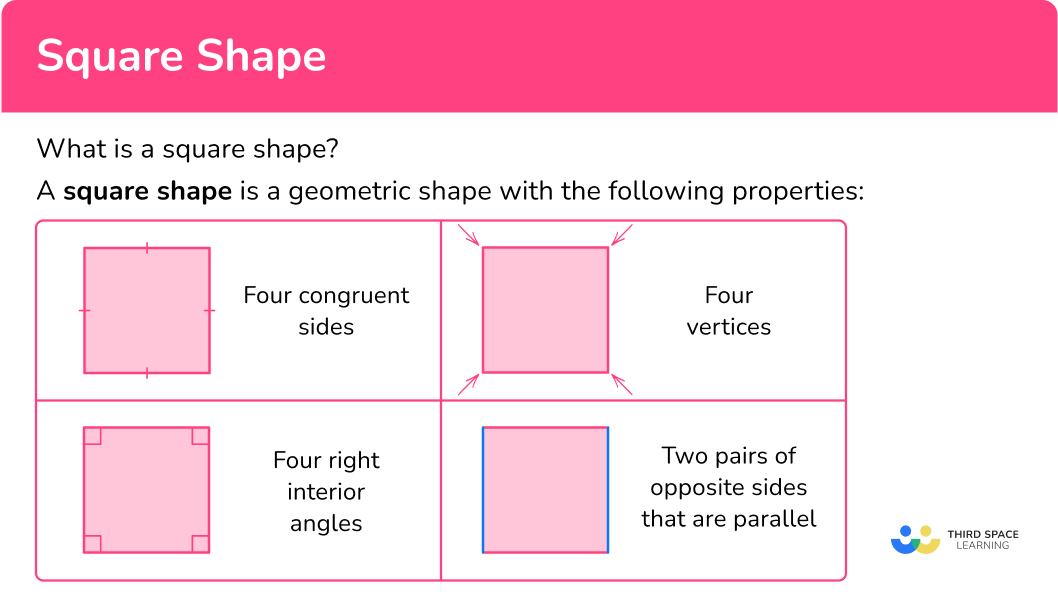## Common Core State Standards

How does this relate to 4 th grade math and 5 th grade math?

• Grade 4 – Geometry (4.G.A.2)
Classify two-dimensional figures based on the presence or absence of parallel or perpendicular lines, or the presence or absence of angles of a specified size. Recognize right triangles as a category, and identify right triangles.

• Grade 5 – Geometry (5.G.B.3)
Understand that attributes belonging to a category of two-dimensional figures also belong to all subcategories of that category. For example, all rectangles have four right angles and squares are rectangles, so all squares have four right angles.

• Grade 5 – Geometry (5.G.B.4)
Classify two-dimensional figures in a hierarchy based on properties.

## How to identify a square shape

In order to identify a square shape:

1. Look for a shape with four equal sides.
2. Decide if the shape has four right angles.

## Square shape examples

### Example 1: identifying a shape

Is the shape a square?

1. Look for a shape with four equal sides.

The shape has four equal sides.

2Decide if the shape has four right angles.

The sides do not form 90 -degree angles.

Two angles are obtuse…

And two angles are acute.

The shape does NOT have four right angles. It is NOT a square.

### Example 2: identifying a shape

Is the shape a square?

The shape has four equal sides.

The sides form 90 -degree angles.

The shape is a square.

### Example 3: identifying a shape

Is the shape a square?

The shape does NOT have four equal sides.

The shape is NOT a square.

### Example 4: identifying a shape

The sides of a shape are: 4{~cm}, 4{~cm}, 4{~cm} and 4{~cm} . Is the shape a square?

The shape has four equal sides.

It is not clear from the description what the angle measurements are. Just because a shape has four equal sides, does not mean the angles are all 90 degrees. More information about the angles is needed to decide if the shape is a square.

### Example 5: finding squares in geometric shapes

How many squares are formed by the geometric shape?

The shapes highlighted have four equal sides.

The sides form 90 -degree angles.

There are two squares in the geometric shape.

### Example 6: finding squares in the real world

How many squares are in the image below?

The shapes in the windows have four equal sides.

Four parts of the window also come together to form a shape with four equal sides.

AND

The sides of the shapes meet at 90 -degree angles.

There are 21 squares in the image.

### Teaching tips for square shape

• Printable worksheets are a great way for students to practice identifying or drawing squares.

• Point out squares in real life and encourage students to look for them as well. This should be an easy task, since there are many real world objects in the shape of a square. Seeing and talking about the properties of squares will help students remember and understand them.

### Easy mistakes to make

• Confusing a square with a rectangle
While a rectangle has many similar features as a square, it is not the same. A rectangle does not have to have four equal sides, but a square does. However, a square fits the definition of a rectangle, so is considered to be both.
For example,

• Mistaking a rhombus for a square
While a rhombus has many similar features as a square, it is not the same. A rhombus does not have to have four right angles, but a square does. However, a square fits the definition of a rhombus, so is considered to be both.
For example,

### Practice square shape questions

1) Which geometric shape is a square?Thinking about the properties, the side lengths of a square are equal, so it cannot be these two shapes:The equal angles of a square all measure 90 -degrees, so it cannot be this shape:This shape is a square:2) Which geometric shape is a square?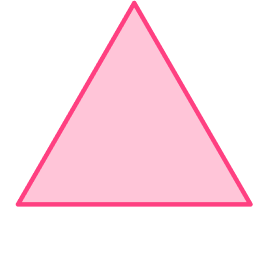Thinking about the properties, a square has four sides, so it cannot be these two shapes: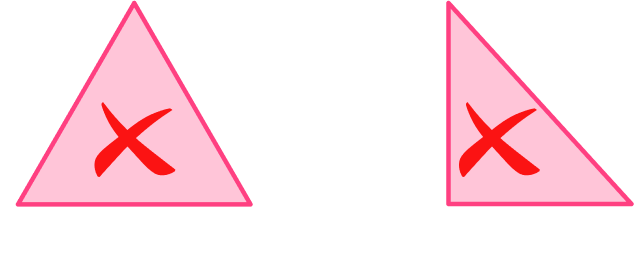Squares also have sides of equal length, so it cannot be this shape:This shape is a square:3) Is this shape a square? Why or why not?Yes, it has 4 right angles.No, it does not have 4 right angles.Yes, it has 4 equal sides.No, it does not have 4 equal sides.A square must have 4 right angles. This shape has two obtuse angles…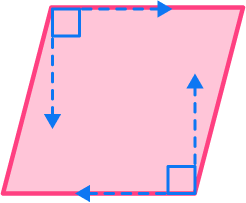and two acute angles.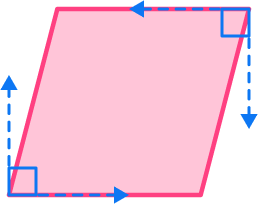It is a rhombus, NOT a square.

4) Which choice describes a square?

length of the sides are all 5{~cm}4 side lengths of 5 inches and 4 right angles4 right angles and side lengths of 6{~ft} and 7{~ft}4 sides and 4 anglesThe description must clearly fit all properties of a square:

• Four congruent sides
• Four vertices
• Four right interior angles
• The opposite sides of a square are parallel

The description “ 4 side lengths of 5 inches and 4 right angles” would be the following shape: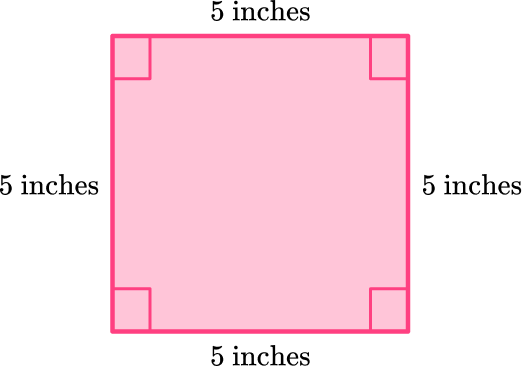This shape fits all the properties, so it is a square.

5) How many squares are formed by the geometric shape?1234The shapes highlighted have four equal sides and four right angles.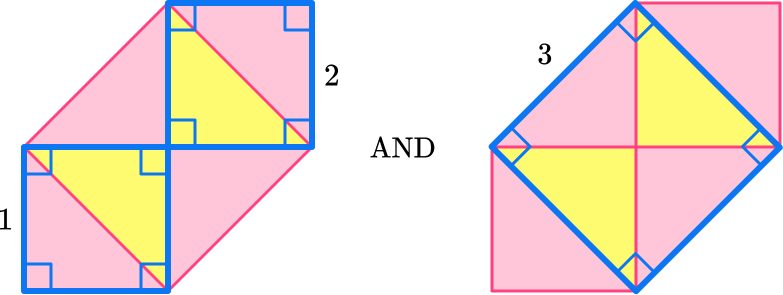There are 3 squares in the geometric shape.

6) How many squares are in the image of a stoplight?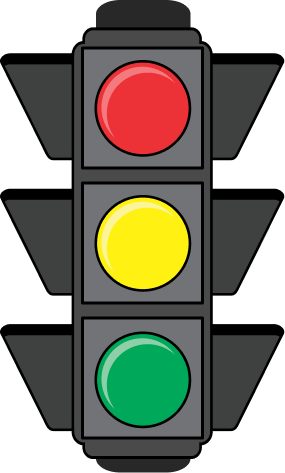1234The shapes highlighted have four equal sides and four right angles.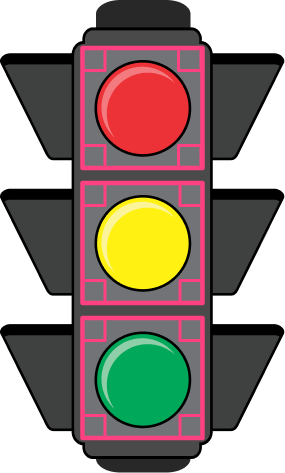There are 3 squares in the stoplight.

## Square shape FAQs

How do you calculate the perimeter of a square?

The perimeter of a square is the distance around the square and can be calculated by multiplying one side length by 4 . Perimeter is measured in units.

Perimeter = side x side x side x side OR 4 x side

How do you calculate the area of a square?

The area of the square is the amount of space inside the square and can be calculated by multiplying two sides together. Area is measured in square units.

Area = side x side OR Side2

What are diagonals of a square?

Diagonals of a square are the lines from one vertex to the opposite vertex. The two lines bisect each other at a 90 -degree angle.

## Still stuck?

At Third Space Learning, we specialize in helping teachers and school leaders to provide personalized math support for more of their students through high-quality, online one-on-one math tutoring delivered by subject experts.

Each week, our tutors support thousands of students who are at risk of not meeting their grade-level expectations, and help accelerate their progress and boost their confidence.